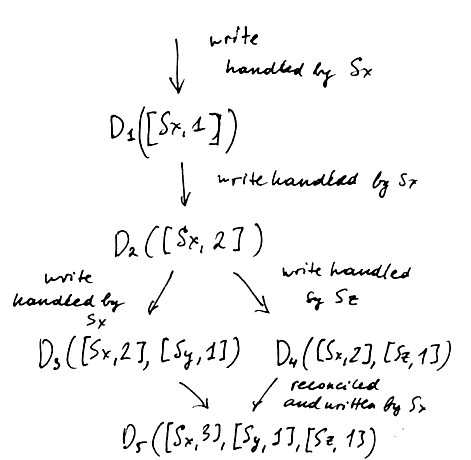# ML Wiki

## Vector Clock

Vector Clock is a popular data structure for ensuring ordering of events in distributes systems. It is often used for achieving Eventual Consistency

def: a vector clock is a tuple $(t_1, ..., t_n)$ of clock values for each node, with $n$ nodes in total.

Notation: for a vector clock $v$, $v[i]$ is the value for node $i$

### Comparison

$v_1 < v_2$ if

• for all $i$: $v_1[i] \leqslant v_2[i]$
• for at least one $j$: $v_1[j] < v_2[j]$

$v_1 < v_2$ implies global time ordering

When data is written to a node $i$, it sets its timestamp $t_i$ to its clock value

### Causation

Suppose we have two events $e_1$ with vector clock $v_1$, and $e_2$ with vector clock $v_2$

$e_1$ happens before $e_2$ if

• $v_1$ < $v_2$

$e_1$ happens concurrently to $e_2$ if

• there exist $i, j$ s.t. $v_1[i] < v_2[i]$ and $v_1[j] > v_2[j]$
• (cannot say if $v_1 < v_2$ or $v_2 < v_1$)

$e_1$ happens after $e_2$ if

• $v_1$ > $v_2$

## Usage in databases

• Every replica in a database keeps a list of number of updates it has seen.
• When an update comes, the replica increases its update counter in the vector clock
• and sends the new clock value with the update to other replicas
• if a read returns a conflicting version, application must reconcile the data and put it back to the database

## Implementation

Since the number of nodes may be big, may prefer to have sparse representation, i.e. to store the vector clock as (node_id, version) pairs

### Voldemort implementation

Here's the Voldemort implementation 

class ClockEntry

• has 2 fields: nodeId and verstion
• method incremented() returns a new ClockEntry with incremented version (same nodeId)

VectorClock

• 2 fields:
• versions: list of ClockEntry classes
• timestamp: time of the last update
• method incrementVersion(nodeId, time)
finds the clock entry for the given node and increments the version; also updates the timestamp
• method incremented(nodeId, time)
same as incrementVerstion, but returns a new VectorClock object
• getMaxVersion() traverses versions and returns the max one
• merge with another VectorClock. Creates a new VectorClock in which
• nodes are merged in sorted order (as in Merge Sort)
• if two nodes have the same nodeId, the max version is used
• method compare returns a value from enum {BEFORE, AFTER, CONCURRENT}

## ExampleSuppose we have 3 servers: $S_x$, $S_y$, $S_z$

1. a client writes $D_1$ at $S_x$: $D_1([S_x, 1])$
2. another client reads $D_1$ and writes back to $D_2$ (also handled by $S_x$)
$D_2([S_x, 2])$ (no conflict and $D_1$ may get garbage collected afterwards)
3. another client reads $D_2$, writes back $D_3$ handled by $S_y$: $D_3([S_x, 2], [S_y, 1])$
4. at the same time someone else also reads $D_2$ and writes back $D_4$ handled by $S_z$
$D_4([S_x, 2], [S_z, 1])$
5. now we have two conflicting versions $D_3$ and $D_4$ (there is no causal relation between these versions, i.e. we cannot decide which one came first - the changes there are different)
both versions must be kept and presented to the client
6. with next read a client gets both $D_3$ and $D_4$, it merges them and writes back.
this is handled by $S_x$: now the version is $D_4([S_x, 3], [S_y, 1], [S_z, 1])$

## Conflicts

How to see if there is a conflict?

 Data 1 Data 2 Conflict? $([S_x, 3], [S_y, 6])$ $([S_x, 3], [S_z, 2])$ Yes $([S_x, 3])$ $([S_x, 5])$ No $([S_x, 3], [S_y, 6])$ $([S_x, 3], [S_y, 6], [S_z, 6])$ No